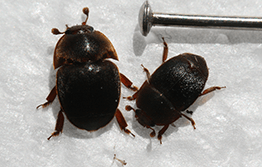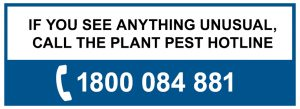```int(43)
bool(false)
int(0)
```
```int(1306)
bool(false)
int(43)
```
```int(2309)
bool(false)
int(43)
```
```int(55)
bool(false)
int(43)
```
```int(1307)
bool(false)
int(43)
```
```int(41)
bool(false)
int(0)
```
```int(60)
bool(false)
int(41)
```
```int(1311)
bool(false)
int(41)
```
```int(1371)
bool(false)
int(41)
```
```int(2576)
bool(false)
int(41)
```
```int(406)
bool(false)
int(41)
```
```int(42)
bool(false)
int(0)
```
```int(1304)
bool(false)
int(42)
```
```int(1303)
bool(false)
int(42)
```
```int(407)
bool(false)
int(42)
```
```int(62)
bool(false)
int(42)
```
```int(51)
bool(false)
int(42)
```
```int(2587)
bool(false)
int(42)
```
```int(3161)
bool(false)
int(42)
```
```int(3163)
bool(false)
int(42)
```
```int(3164)
bool(false)
int(42)
```
```int(3165)
bool(false)
int(42)
```
```int(3166)
bool(false)
int(42)
```
```int(3167)
bool(false)
int(42)
```
```int(3168)
bool(false)
int(42)
```
```int(3169)
bool(false)
int(42)
```
```int(3170)
bool(false)
int(42)
```
```int(3171)
bool(false)
int(42)
```
```int(3172)
bool(false)
int(42)
```
```int(3173)
bool(false)
int(42)
```
```int(3174)
bool(false)
int(42)
```
```int(2951)
bool(false)
int(0)
```
```int(2952)
bool(false)
int(2951)
```
```int(2953)
bool(false)
int(2951)
```
```int(2954)
bool(false)
int(2951)
```
```int(2955)
bool(false)
int(2951)
```The small hive beetle can significantly damage beehives and stored hive material and contribute to hive death. The beetle feeds on live brood and honey and its excrement can contaminate honey and cause it to ferment.

It’s found in Queensland, New South Wales and Western Australia. If you think you have seen small hive beetle anywhere else in Australia, call 1800 084 881.

The adult beetle lays clumps of small, whitish eggs in the beehive. Small, cigar-shaped, cream-coloured larvae hatch and, along with the adults, feed on stored honey and pollen in the hive.The beetle is spread by the movement of bees and beehives and through direct flight of the adults.